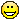# How to continuously control line thickness in plotting functions?

Posted 9 years ago
5875 Views
|
5 Replies
|
25 Total Likes
|
 I know that I can control color and opacity of lines in plotting functions  like in the example below. I also would like the line to change its thickness smoothly as we move in the positive x-direction. Is it possible to do via options to Plot? If not, - what could be the easiest approaches? Plot[Sin[x^2], {x, -3, 3}, PlotStyle -> Thickness[.04], PlotPoints -> 50,   ColorFunction -> Function[{x, y}, Directive[ColorData["Rainbow"][x], Opacity[Sin[10 y + 15 x]]]]]Answer
5 Replies
Sort By:
Posted 9 years ago
 This whole thread demonstrates mostly for better the stupifying generality of Mathematica. Awesome.Answer
Posted 9 years ago
 Min, that is an awful abuse of Mesh.  I like it.Answer
Posted 9 years ago
 Using MeshShading will also work but not as good as ParametricPlot and using Filling but it doesn't change the function to use and original input.Plot[Sin[x^2], {x, -3, 3}, Mesh -> {Range[-3, 3, 6/100]}, MeshStyle -> None,MeshShading -> (Directive[Hue[Rescale[#, {-3, 3}, {0, 1}]],AbsoluteThickness[Rescale[#, {-3, 3}, {1, 20}]]] & /@Range[-3, 3, 6/100]), PlotRangePadding -> Scaled[0.05]]Answer
Posted 9 years ago
 It crossed my mind that in some situations we can take advantage of Filling:Table[Plot[Evaluate[  Flatten[{#, # + amp (x + 3.5), # - amp (x + 3.5)} & /@ {Cos[x^2], .3 Sin[10 x]}]], {x, -3, 3},  PlotPoints -> 50, Filling -> {3 -> {2}, 5 -> {6}}, PlotStyle -> {None, Gray, Gray, None, Gray, Gray},  ColorFunction -> Function[{x, y}, Directive[ColorData["Rainbow"][x], Opacity[x]]],  AspectRatio -> 1/4, ImageSize -> 500], {amp, .005, .15, .05}]The controlled thickness is only vertical in this case. It will not work in some other cases, for example ParametricPlot does not have Filling option at all. But, in ParametricPlot case, for example, we can use the function itself, because it can show bounded areas:ParametricPlot[{(v + 1) u Cos[u], (v + 1) u Sin[u]}, {u, 0, 6 Pi}, {v, 0.5, 1}, Mesh -> None, MaxRecursion -> 4, Axes -> False, BoundaryStyle -> GrayLevel[.9],  ColorFunction -> Function[{x, y, u, v}, Directive[ColorData["Rainbow"][u v], Opacity[v]]]]Answer
Posted 9 years ago
 Not a general solution, but this works some times:ParametricPlot3D[{{x, Sin[x^2], x}, {x, Cos[x^2], x}}, {x, 0 - 3, 3},PlotStyle -> {{Opacity[0.5], Tube[x/2000]}, {Opacity[0.3], Tube[x/2000]}},ViewPoint -> {0, 0, 1000}, PlotRange -> All, ColorFunction -> "Rainbow"]npts = 12;ParametricPlot3D[{{Cos[2 t], Sin[2 t], Cos}, {Sin[2 t], Cos[2 t], Sin}}, {t, 0, 2 Pi},  PlotStyle -> {{Red, Tube[{0.1 Sin[t/(2 Pi)], 0.1}, PlotPoints -> npts, Frame -> "Frenet"]},                {Yellow, Tube[{0.1 Sin[t/(2 Pi)], 0.1}, Frame -> "Frenet", PlotPoints -> npts]}},  PlotRange -> All, MaxRecursion -> 0, PlotPoints -> 200]Answer